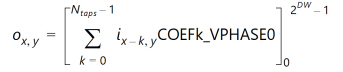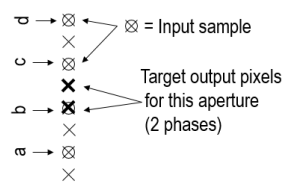# Convert 4:2:0 to 4:2:2 - 2.4 English

## Video Processing Subsystem Product Guide (PG231)

Document ID
PG231
Release Date
2022-10-19
Version
2.4 English

This conversion is a 1:2 vertical interpolation operation, implemented using a 2-phase polyphase FIR filter. One of the two output pixels is co-sited with one of the input sample. The ideal output is achieved simply by replicating this input sample.

To evaluate output pixel o x,y, the FIR filter in the core convolves COEFk_VPHASEp y , where k is the coefficient index, p y is the interpolation phase, i x,y are pixels from the input image, and [ ] M m represents rounding with clipping at M, and clamping at m. DW is the Data Width or number of bits per video component. Ntaps is the number of filter taps.

Equation 3-12In phase 1, COEF00_VPHASE1 is the coefficient applied to the most recent input sample in the filter. This Figure illustrates coefficient use for a four tap filter example, with simplified nomenclature a= COEF00_VPHASE1, b= COEF01_VPHASE1, c= COEF02_VPHASE1, and d= COEF03_VPHASE1.

 X-Ref Target - Figure 3-15The predefined filters use the coefficients [0.5 0.5] to interpolate one of the output samples. The other output sample is a replication of the input sample.